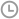•发布时间：2018-07-26 09:26:46
• |
• 出处：PDF猫
• |
• 阅读量：39

A：下面介绍如何使用数组公式来实现目的。为便于理解，我们先使用一些中间结果，然后组合成最终的数组公式。=MID(B2,ROW(INDIRECT("1:"& LEN(B2))),1)

=1*MID(B2,ROW(INDIRECT("1:"& LEN(B2))),1)

=ISERROR(1*MID(B2,ROW(INDIRECT("1:"& LEN(B2))),1))

=MATCH(TRUE,ISERROR(1*MID(B2,ROW(INDIRECT("1:"& LEN(B2))),1)),0)

=MID(B2,MATCH(TRUE,ISERROR(1*MID(B2,ROW(INDIRECT("1:"& LEN(B2))),1)),0),LEN(B2))

=MATCH(FALSE,ISERROR(1*MID(G2,ROW(INDIRECT("1:"& LEN(B2))),1)),0)

=IF(ISNA(H2),B2,"")

=IF(ISNA(MATCH(FALSE,ISERROR(1*MID(MID(B2,MATCH(TRUE,ISERROR(1*MID(B2,ROW(INDIRECT("1:"& LEN(B2))),1)),0),LEN(B2)),ROW(INDIRECT("1:" &LEN(B2))),1)),0)),B2,"")PDF猫转换器

PDF猫转换器